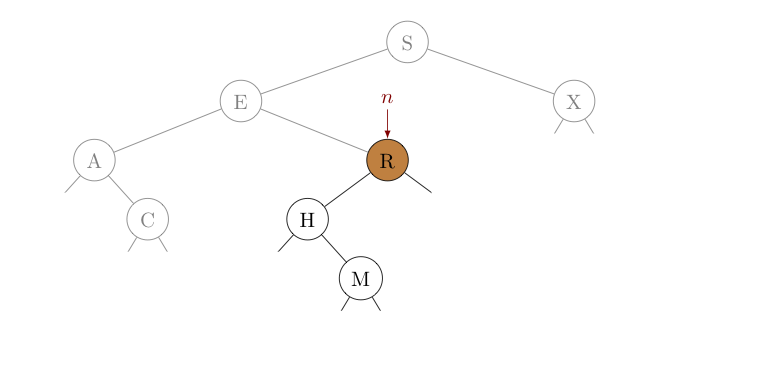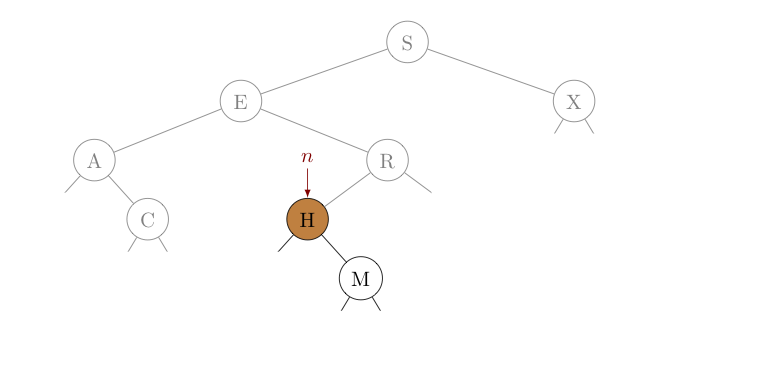## Searching and Inserting in a Binary Search Tree

As previously mentioned, we can use a binary search tree as a symbol table to store key-value pairs. As such, it will need to support the operations symbol tables require.

Recalling our previously described SymbolTable interface, that includes a get() method for searching for a key so that its corresponding value can be returned, and a put() method for inserting (or updating) key-value pairs in the tree:

public interface SymbolTable<Key, Value> {

Value get(Key key);              // returns the value paired with given key

void put(Key key, Value value);  // inserts a key-value pair into the table,
// or updates the value associated with a key if it
...
}


As we consider the algorithms and implementations behind these two methods -- let us suppose we start with the following skeleton for our BinarySearchTree class:

public class BinarySearchTree<Key extends Comparable<Key>,Value>
implements SymbolTable<Key,Value> {

private class Node {
private Key key;     // the key
private Value val;   // the value

private Node left;   // a reference to the left child of this node
private Node right;  // a reference to the right child of this node

...

public Node(Key key, Value val) {   // a constructor to make
this.key = key;                 // Node creation simple
this.val = val;
}
}

private Node root;  // a reference to the root node of the tree -- through
// which, references to all other nodes can be found

...
}


A couple of things above bear mentioning. First, note that we have insisted that our keys implement the Comparable interface. This, of course, allows us to compare the keys in our tree with any keys for which we might be searching.

Related to this -- and as a required property of binary search trees -- we assume they are kept in symmetric order, by key. Recall for a tree to be in symmetric order, the keys in all of the left descendents of any given node must be less than that node's key, and the keys in all of the right descendents of any given node must be greater than that node's key.

With this in mind, let's take a closer look at the get() method, which retrieves the value associated with a given key...

#### The get() method

Note that each node has several instance variables associated with it, but the entire BinarySearchTree class has only one -- a reference to the node identified as root. To return the value associated with key, we'll need to find a reference to the node containing key. However, if all we have is a reference to root, how do we get the reference we need?

As a specific example, consider the tree drawn below. Suppose we seek the node with key $H$. Create a new reference $n$ identical to our tree's root (which references node $S$). Then compare $H$ (the key sought) with the key $S$ at $n$.Noting that $H \lt S$, if $H$ is present in this tree, the symmetric order of the tree requires it is in the subtree rooted at $E$, the left child node $n$. As such, we update $n$ to reference its own left child, $E$. In the picture below, the portion of the tree eliminated from consideration has been "grayed out". Then, we start over with this subtree -- we compare $H$ (the key sought) with the key $E$ at $n$.Noting that $E \lt H$, if $H$ is present in this tree, the symmetric order this time requires it to be in the subtree rooted at $R$, the right child of $n$. Consequently, we update $n$ to reference its own right child, $R$. Notice, we are quickly reducing the number of places we need to look to find $H$ -- as can be seen by the amount of the tree now "grayed out".We again compare $H$ (the key sought) with the key at $n$, and seeing $H \lt R$, we update $n$ to reference its left child.Of course, when we compare $H$ this time with the key at $n$, we see they are equal -- we found the node with $H$ as its key! We can return the value of this node using $n$ and are done. When a search is successful in this way, we call it a search hit.

What would have happened if we were looking for a key that wasn't in the tree instead? Suppose we were searching above for $G$, instead of $H$. Noting that $G \lt S$, $G \gt E$, and $G \lt R$, we would have made the same first three choices for updating $n$, as we did above. However, as $G \lt H$, we would have then updated $n$ to the left child of $H$, which is null. $G$ clearly can't be in an empty subtree, so we can be confident $G$ does not appear in the tree at all and return null to the client as a result. Perhaps predictably, we call the resulting failed search for a key a search miss.

We can easily implement the above search process either iteratively and recursively. The iterative method is simpler, but the recursive approach will reveal an important strategy. With this in mind, we'll consider both techniques. The iterative version is shown below, and hopefully now fairly self-explanatory.

public Value get(Key key) {

Node n = root;

while (n != null) {
int cmp = key.compareTo(n.key);

if (cmp < 0)        // key < n.key
n = n.left;

else if (cmp > 0)   // key > n.key
n = n.right;

else                // key == n.key   (found it!)
return n.val;
}

}


Think about the number of comparisons of keys made in this process. Presuming the root is not null (in which case no comparisons are made), we make one comparison for the root node, and an additional comparison for each level up to and including the level at which we find (or don't find) the key sought.

So the number of comparisons made is one more than the depth of the node. This means the worst-case number of comparisons is one more than the height of the tree. For perfectly balanced binary search trees of $n$ nodes, recall this is $\sim \log_2 n$. Consequently, retrieving a value for a given key in a perfectly balanced binary search tree incredibly fast!

Before showing a recursive version of get(), a few observations are in order.

First, recall that with each comparison made above, we either:

• found the key sought;
• discovered the key wasn't in the tree to begin with; or
• reduced the problem to finding the key sought in some (smaller) subtree.
The first two establish base cases, while the last establishes the recursive case.

Since we are recursing on subtrees rooted at different nodes, we will need to pass the reference for each subtree root as part of our recursive call. This creates a bit of a problem, though. We won't be able to get by with just get(Key key) -- we'll need a method that looks like get(Node n, Key key). That said, we also must not expose the inner Node class to the client! It could easily be the case that the client is using the class defining our binary search tree strictly as a symbol table. In such contexts, the client is expecting to deal with some Key and Value classes -- but that's it. Remember, classes should work as "black-boxes" -- promising to implement the public methods of their APIs, but never revealing how these methods do what they do. Consequently, everything about nodes needs to stay hidden.

There is a simple fix for this problem. We simply make two methods. We make a public-facing get(Key key) method as well as a private, recursive method get(Node n, Key key). The public method is written so that all it really does is call the recursive method to do the "heavy lifting". Note, in this first call to the recursive method, the node passed needs to be root.

With all that in mind, here's how we can use recursion to define get():

public Value get(Key key) {
return get(root, key);
}

public Value get(Node n, Key key) {

if (n == null)

int cmp = key.compareTo(n.key);

if (cmp < 0)
return get(n.left, key);  // key < n.key

else if (cmp > 0)
return get(n.right, key); // key > n.key

else
return n.val;             // key == n.key  (found it!)
}


Notably, the cost of this implementation to get() is slightly higher than our previous implementation, due to the recursive overhead.

#### The put() method

Understanding how to implement the put() method recursively will make later operations (e.g., delete(Key key) easier, so let us describe that process here, and leave the iterative version of this method for an exercise.

In the put(Key key, Value val) method below, which inserts (or updates) the tree with the given key-value pair, we use the same "split-into-two-methods" tactic seen in the recursive get() method earlier.

Also similar to what we did in get(), we find the location for the insertion (or update) by comparing each node key examined to the one to be inserted/updated, and then recursing on the left or right subtree as appropriate. The details of the base and recursive cases change slightly, however:

• The smallest subtree would be an empty tree (i.e., a tree with a null root. In this base case, we want to return a tree with a single node corresponding to the given key-value pair. So, we construct a new node, filling it with our given key and value, and then return a reference to that node. Remember, every tree we have is referenced by its single top-most node -- so we can think of this as returning an entire (but tiny) tree.

• For any other key, we can compare the root's key with the key to insert. There are three possibilities to consider:

• If the key to insert is smaller than the root's key, we know that whatever change must happen to put the new key-value in the tree will have to happen in the subtree rooted at the root's left child. In this case, we re-assign the root's left child to be the result of putting the given key-value pair in the left subtree. Again, remember that by "result" here, we mean the single node reference to a tree where the new key-value pair has been inserted.

• If the key to insert is larger than the root's key, the needed change must instead happen in the subtree rooted at the root's right child. So we similarly re-assign the right child to be the result of "putting" this key-value pair in this right subtree.

• As the last case to consider, If the key to insert agrees with the root's key, we must be updating this root node. This is actually another base case -- assign the given value to n.val and call it a day.

Here's the code:

public void put(Key key, Value val) {
root = put(root, key, val);
}

private Node put(Node n, Key key, Value val) {

if (n == null)  {                      // (base case, insert)
Node newNode = new Node(key, val);
return newNode;
}

int cmp = key.compareTo(n.key);

if (cmp < 0)                          // key < n.key  (recurse on left subtree)
n.left = put(n.left, key, val);

else if (cmp > 0)                     // key > n.key  (recurse on right subtree)
n.right = put(n.right, key, val);

else                                  // key == n.key (base case, overwrite)
n.val = val;

return n;
}


Navigating the path to the insertion point requires a number of comparisons equal to one more than the level of the key-value pair after it has been inserted, with the worst-case again being a number of comparisons one more than the height of the tree. Thus, insertion into a binary search tree is also fast, with comparison cost $\sim \log_2 n$ for a perfectly balanced tree of $n$ nodes.